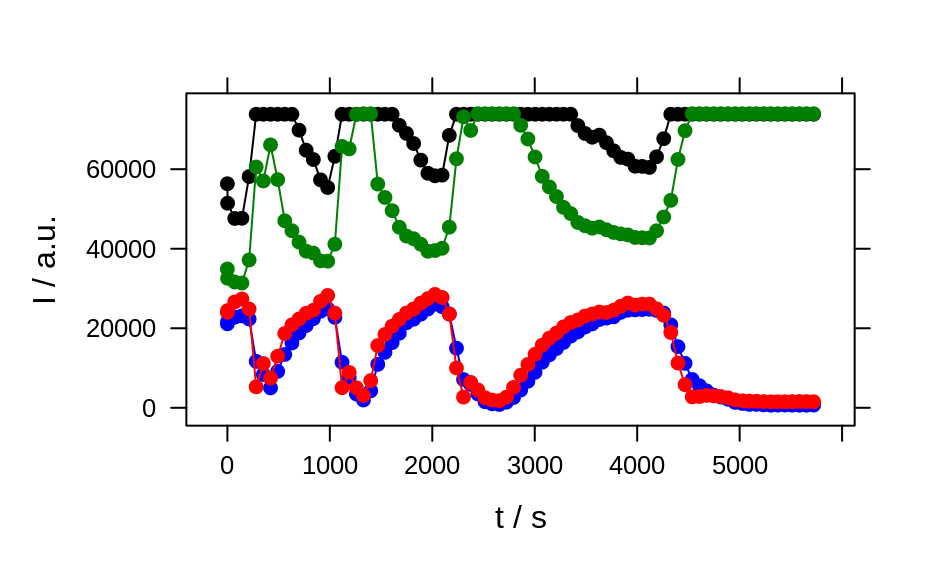Calibration- and timeseries plots, depth-profiles and the like plotc plots intensities of a hyperSpec object over another dimension such as concentration, time, or a spatial coordinate.

plotc(
object,
model = spc ~ c,
groups = NULL,
func = NULL,
func.args = list(),
...
)

Arguments

object

the hyperSpec object

model

the lattice model specifying the plot

groups

grouping variable, e.g. .wavelength if intensities of more than one wavelength should be plotted

func

function to compute a summary value from the spectra to be plotted instead of single intensities

func.args

further arguments to func

...

further arguments to lattice::xyplot().

Details

If func is not NULL, the summary characteristic is calculated first by applying func with the respective arguments (in func.args) to each of the spectra. If func returns more than one value (for each spectrum), the different values end up as different wavelengths.

If the wavelength is not used in the model specification nor in groups, nor for specifying subsets, and neither is func given, then only the first wavelength's intensities are plotted and a warning is issued.

The special column names .rownames and .wavelength may be used.

The actual plotting is done by lattice::xyplot().

C. Beleites

Examples

## example 1: calibration of fluorescence
plotc(flu) ## gives a warning
#> Warning: Intensity at first wavelengh only is used.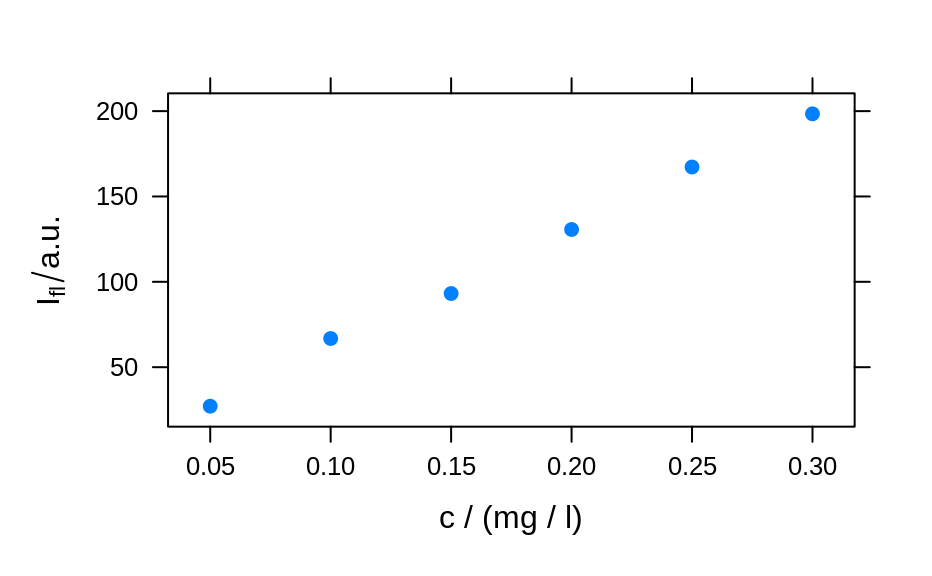plotc(flu, func = mean)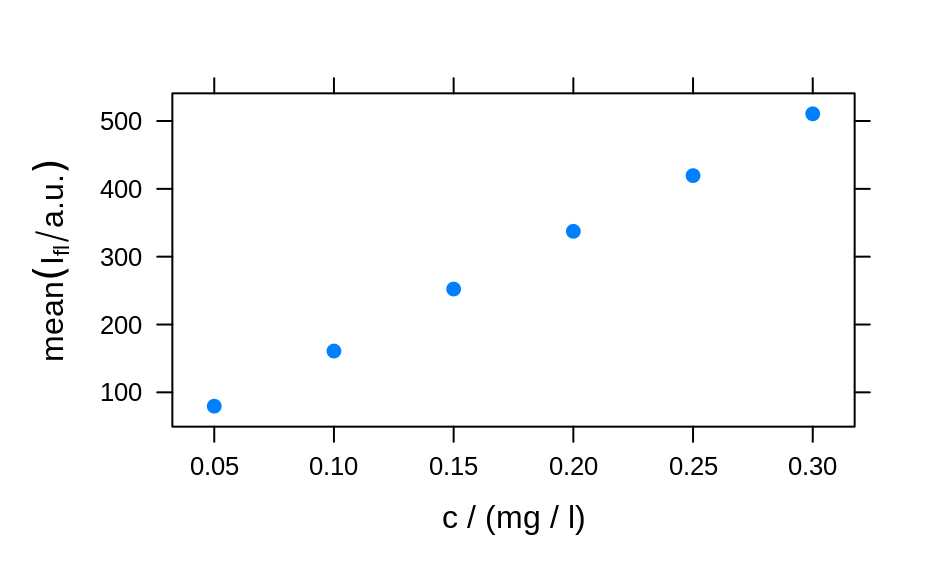plotc(flu, func = range, groups = .wavelength)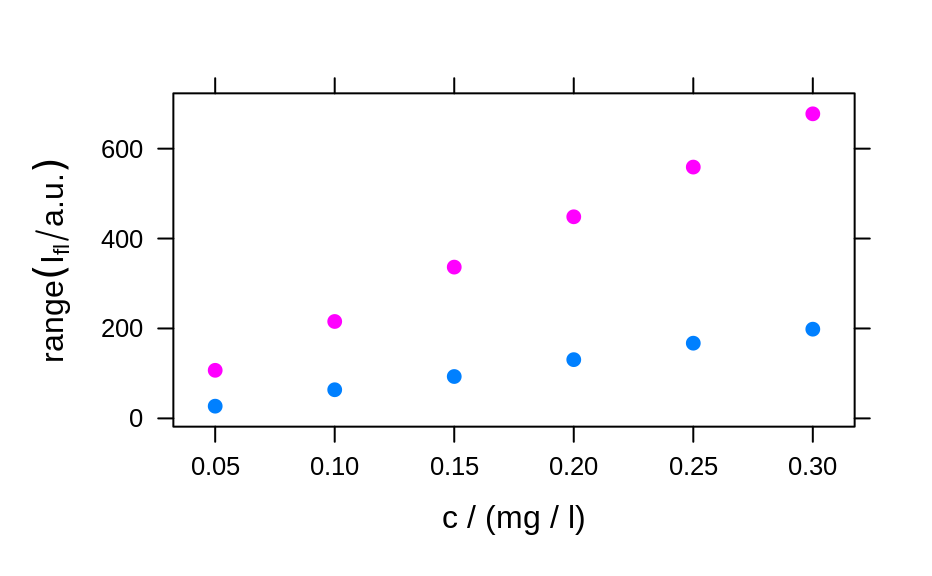plotc(flu[, , 450], ylab = expression(I["450 nm"] / a.u.))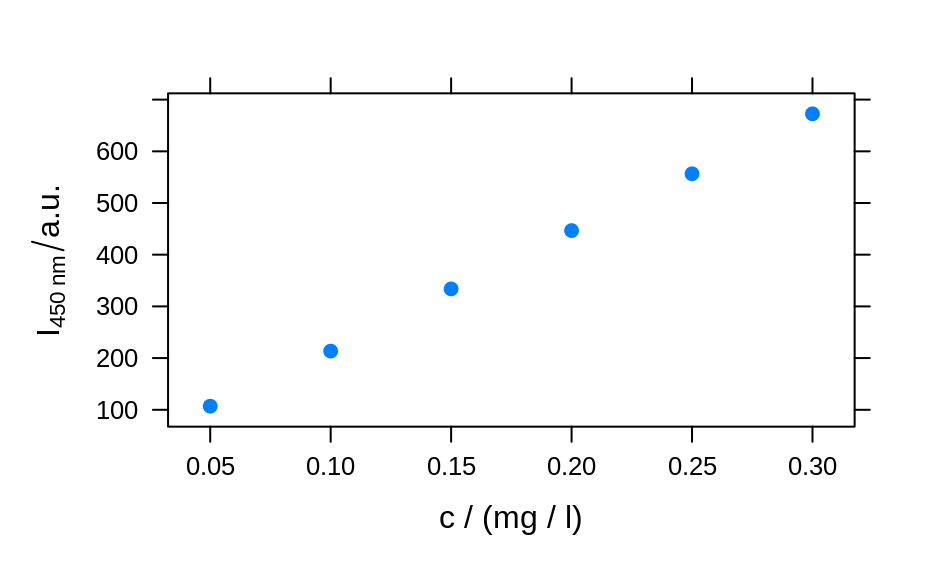calibration <- lm(spc ~ c, data = flu[, , 450]$.) summary(calibration) #> #> Call: #> lm(formula = spc ~ c, data = flu[, , 450]$.)
#>
#> Residuals:
#>       1       2       3       4       5       6
#>  2.1918 -4.6846  2.1696  1.6005 -1.9306  0.6533
#>
#> Coefficients:
#>             Estimate Std. Error t value Pr(>|t|)
#> (Intercept)   -8.666      2.876  -3.013   0.0394 *
#> c           2268.482     14.769 153.596 1.08e-08 ***
#> ---
#> Signif. codes:  0 ‘***’ 0.001 ‘**’ 0.01 ‘*’ 0.05 ‘.’ 0.1 ‘ ’ 1
#>
#> Residual standard error: 3.089 on 4 degrees of freedom
#> Multiple R-squared:  0.9998,	Adjusted R-squared:  0.9998
#> F-statistic: 2.359e+04 on 1 and 4 DF,  p-value: 1.078e-08
#>
plotc(flu[, , 450], type = c("p", "r"))conc <- list(c = seq(from = 0.04, to = 0.31, by = 0.01))
ci <- predict(calibration, newdata = conc, interval = "confidence", level = 0.999)

panel.ci <- function(x, y, ...,
conc, ci.lwr, ci.upr, ci.col = "#606060") {
panel.xyplot(x, y, ...)
panel.lmline(x, y, ...)
panel.lines(conc, ci.lwr, col = ci.col)
panel.lines(conc, ci.upr, col = ci.col)
}

plotc(flu[, , 450],
panel = panel.ci,
conc = conc\$c, ci.lwr = ci[, 2], ci.upr = ci[, 3]
)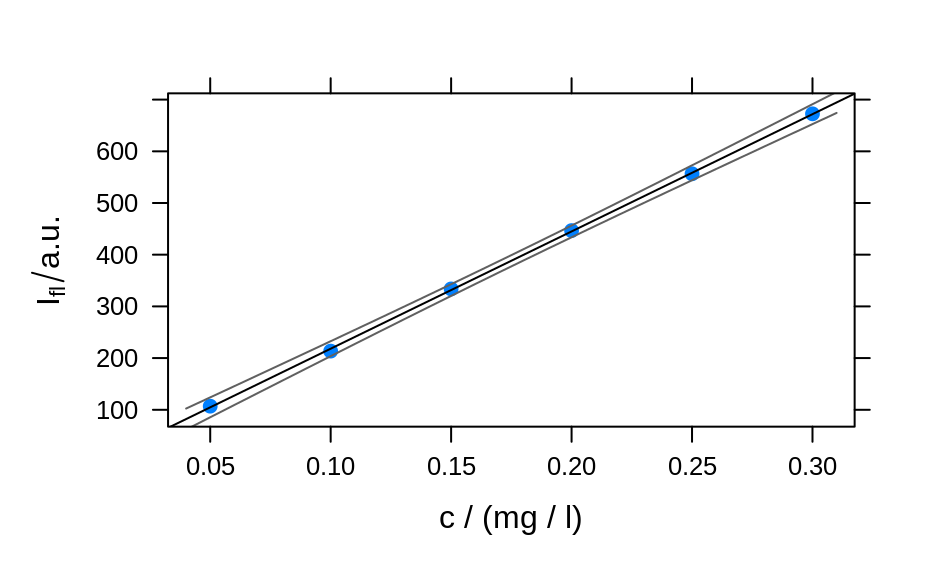## example 2: time-trace of laser emission modes
cols <- c("black", "blue", "#008000", "red")
wl <- i2wl(laser, c(13, 17, 21, 23))

plot_spc(laser, axis.args = list(x = list(at = seq(404.5, 405.8, .1))))
for (i in seq_along(wl)) {
abline(v = wl[i], col = cols[i], lwd = 2)
}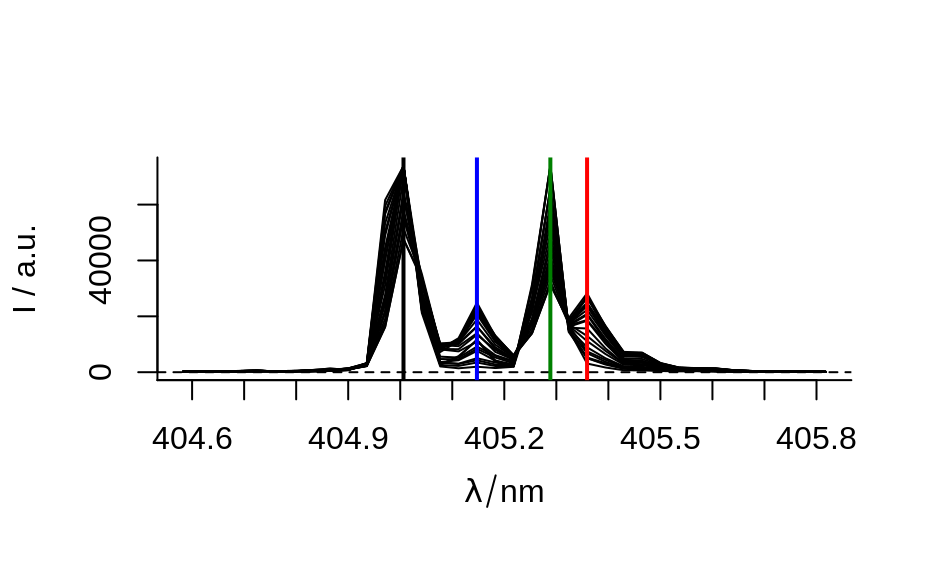plotc(laser[, , wl], spc ~ t,
groups = .wavelength, type = "b",
col = cols
)